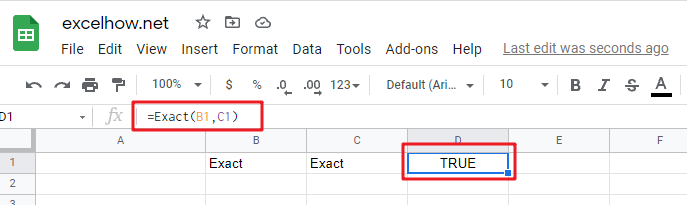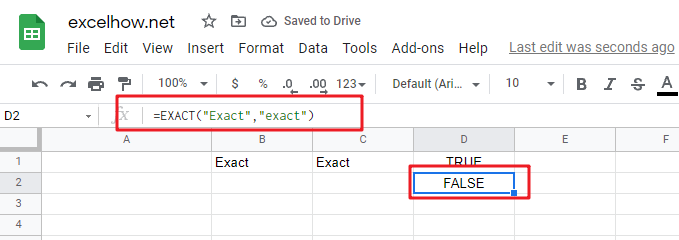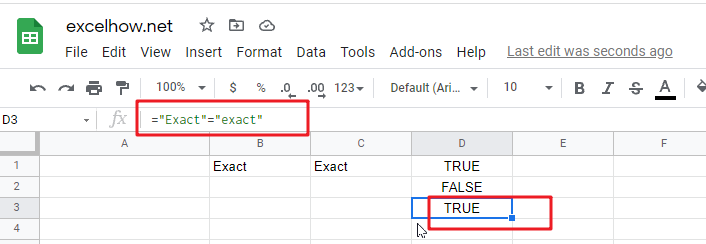# ExcelHow

This post will guide you how to use Google Sheets EXACT function with syntax and examples.

## Description

The Google Sheets EXACT function compares if two text strings are the same and returns TRUE if they are the same, Or, it will return FALSE.

The EXACT function can be used to test whether two given text strings are identical in Google Sheets.

The EXACT function is a build-in function in Google Sheets and it is categorized as a Text Function.

## Syntax

The syntax of the EXACT function is as below:

= EXACT (text1,text2)

Where the EXACT function arguments are:

• Text1 and Text2-This is a required argument.  Two text strings that you want to compare.

Text1 is the first string to compare; Text2 is the second string to compare. If the two strings are the same, it returns TRUE, otherwise, it returns FALSE.

The EXACT function must be specified two arguments, text1 and text2, which should be a valid text string values. If these text string values are entered into the formula, you need to enclose these values in double quotes in google sheets formula.

## Example

The below example will show you how to use google sheets EXACT Text function to check whether the two text string values are identical.

#1 To compare if the text strings in both B1 and C1 cells are the same, just using formula:

` =EXACT(B1,C1)`#2  To compare two hardcoded text strings, using the following EXACT formula in google sheets:

`=EXACT("Exact","exact")`#3 To compare two text string without case sensitive, and you can use the equal sign operation in a formula, type:

`="Exact"="exact"`Notes:

• The EXACT function is case-sensitive.
• The EXACT function would return a Boolean value (TRUE or FALSE)
• The EXACT function requires the two arguments to have the exact same characters, including identical text case and identical special characters (spaces or hidden characters)